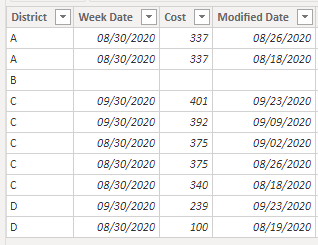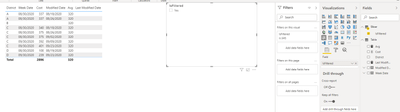cancel
Showing results for
Did you mean:

Fabric is Generally Available. Browse Fabric Presentations. Work towards your Fabric certification with the Cloud Skills Challenge.Helper II

I am trying to do a few things here with my data.

The first is to show the last value modified for the current month when a filter is selected. This I have figured out.

The second (my issue), is when a filter is not selected, to average the last modified values across all districts for the current month. I need it to average the dates in yellow. It is currently averaging the values in orange.``````VAR MD =
CALCULATE(
MAX('DM Labor'[Modified]),ALLEXCEPT('DM Labor','DM Labor'[District]))
VAR LF =
CALCULATE(
AVERAGE('DM Labor'[Labor/Fleet]),FILTER('DM Labor','DM Labor'[Modified]=MD),FILTER('DM Labor',MONTH('DM Labor'[Weekof])=MONTH(LASTDATE(Dates[Date]))))/1000
VAR LF1=
CALCULATE(AVERAGE('DM Labor'[Labor/Fleet]),
FILTER('DM Labor',MONTH('DM Labor'[Weekof])=MONTH(LASTDATE(Dates[Date]))))/1000
VAR LFCM = CALCULATE(
IF(ISFILTERED('Maintenance Plants'[District]),LF,LF1))
RETURN
IF(ISBLANK(LFCM),"NA",LFCM)``````
1 ACCEPTED SOLUTIONHelper II

This was my last soul to get the unique value to show on a card.

``````VAR MD =
CALCULATE(
MAX('DM Labor'[Modified]),FILTER(ALL('DM Labor'),YEAR('DM Labor'[Modified])=YEAR(TODAY())&&MONTH('DM Labor'[Modified])=MONTH(TODAY())))
VAR Tab =
ALL('DM Labor'),
'DM Labor'[District],
"last date",
MAX('DM Labor'[Modified])),
"result",
CALCULATE(
AVERAGE('DM Labor'[Labor/Fleet]),
FILTER(
ALL('DM Labor'),
'DM Labor'[District]=EARLIER('DM Labor'[District])&&
'DM Labor'[Modified]=EARLIER([last date])&&MONTH('DM Labor'[Weekof])=MONTH(TODAY()))))
VAR LFCM = AVERAGEX(Tab,[result])/1000
Return
IF(ISBLANK(LFCM),"NA",LFCM)``````
2 REPLIES 2Community Support

Based on your description, I created data to reproduce your scenario. The pbix file is attached in the end.

Table:Slicer:You may create two measures as below.

``````Last Modified Date =
var _maxdate =
CALCULATE(
MAX('Table'[Modified Date]),
FILTER(
ALL('Table'),
YEAR([Modified Date])=YEAR(TODAY())&&
MONTH([Modified Date])=MONTH(TODAY())
)
)
return
IF(
ISFILTERED(Slicer[IsFiltered]),
_maxdate
)

Avg =
var _maxdate =
CALCULATE(
MAX('Table'[Modified Date]),
FILTER(
ALL('Table'),
YEAR([Modified Date])=YEAR(TODAY())&&
MONTH([Modified Date])=MONTH(TODAY())
)
)
var tab =
SUMMARIZE(
ALL('Table'),
'Table'[District],
"LastDate",
MAX('Table'[Modified Date])
),
"Result",
CALCULATE(
SUM('Table'[Cost]),
FILTER(
ALL('Table'),
'Table'[District]=EARLIER('Table'[District])&&
'Table'[Modified Date]=EARLIER([LastDate])
)
)
)
return
IF(
NOT( ISFILTERED(Slicer[IsFiltered]) ),
AVERAGEX(
FILTER(
tab,
[LastDate]=_maxdate
),
[Result]
)
)``````

Result:Best Regards

Allan

If this post helps, then please consider Accept it as the solution to help the other members find it more quickly.Helper II

This was my last soul to get the unique value to show on a card.

``````VAR MD =
CALCULATE(
MAX('DM Labor'[Modified]),FILTER(ALL('DM Labor'),YEAR('DM Labor'[Modified])=YEAR(TODAY())&&MONTH('DM Labor'[Modified])=MONTH(TODAY())))
VAR Tab =
ALL('DM Labor'),
'DM Labor'[District],
"last date",
MAX('DM Labor'[Modified])),
"result",
CALCULATE(
AVERAGE('DM Labor'[Labor/Fleet]),
FILTER(
ALL('DM Labor'),
'DM Labor'[District]=EARLIER('DM Labor'[District])&&
'DM Labor'[Modified]=EARLIER([last date])&&MONTH('DM Labor'[Weekof])=MONTH(TODAY()))))
VAR LFCM = AVERAGEX(Tab,[result])/1000
Return
IF(ISBLANK(LFCM),"NA",LFCM)``````Announcements#### Power BI Monthly Update - November 2023

Check out the November 2023 Power BI update to learn about new features.#### Fabric Community News unified experience

Read the latest Fabric Community announcements, including updates on Power BI, Synapse, Data Factory and Data Activator.#### The largest Power BI and Fabric virtual conference

130+ sessions, 130+ speakers, Product managers, MVPs, and experts. All about Power BI and Fabric. Attend online or watch the recordings.Top Solution Authors
Top Kudoed Authors
Users online (3,823)